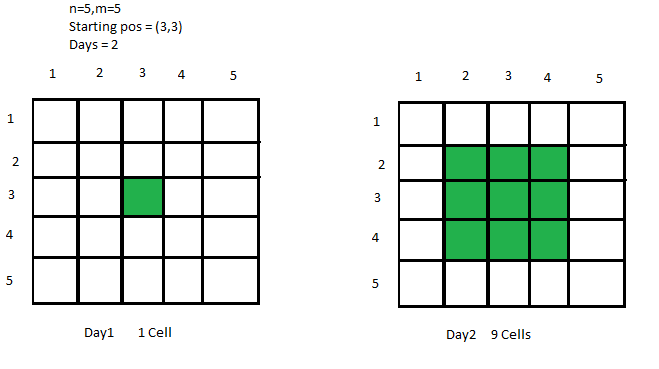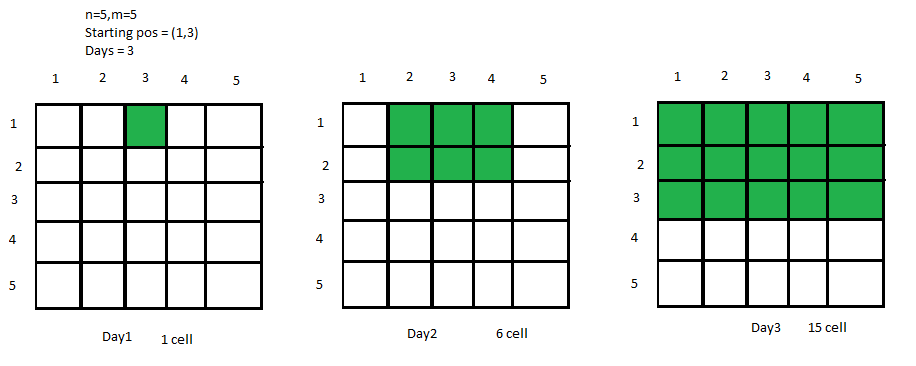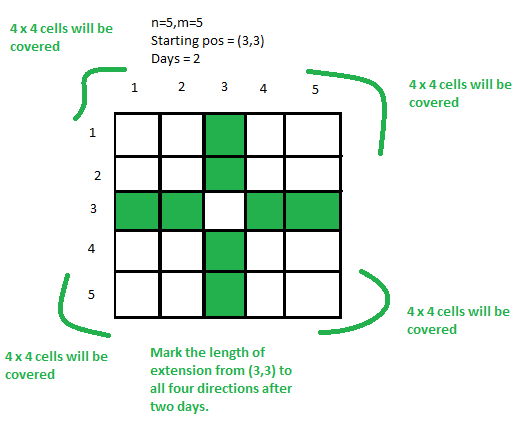# Total number of cells covered in a matrix after D days

Given an N * M matrix and a starting position (X, Y) of a virus, the task is to find out the number of covered cells after D days, if the virus spreads from its current cell to its adjacent cells every day.Examples:

Input: N = 5, M = 5, X = 1, Y = 3, D = 3Output: 15
Explanation:
We can clearly see from the picture that 15 cells are covered after 3 days.
Input: N = 10, M = 10, X = 7, Y = 8, D = 4
Output: 42
Explanation:
On making an N * M matrix and filling the adjacent cells for 4 days we will get 42 covered cells.

Approach:
To solve the problem mentioned above we have to observe clearly that from a starting cell, we just need to find out the extension of cells towards top, right, bottom and left after D days. Then calculate the total cells inside every quadrilateral of cells formed and add them all.
Therefore, the total answer will be the sum of all cells of quadrilaterals after D days + the total cells that are along the top, right, down, left, and 1 (for Starting cell) keeping in consideration the boundaries of the quadrilateral.
Below is the condition for extension in all four directions:

Extension upto Top -> min(D, X-1)
Extension upto Down -> min(D, N-X)
Extension upto Left -> min(D, Y-1)
Extension upto Right -> min(D, M-Y)

Look at the image below for clear understanding:Now multiply Top * Left, Top * Right, Down * Left, Down * Right and add all of them and also add the total cells along the line of 4 directions. We also add 1(for starting cell) to get the resultant cells.
Below is the implementation of above approach:

## C++

 `// C++ implementation to find the ` `// Total number of cells covered ` `// in a matrix after D days `   `#include ` `using` `namespace` `std; `   `// Function to return the total ` `// infected cells after d days ` `int` `solve(``int` `n, ``int` `m, ``int` `x, ` `        ``int` `y, ``int` `d) ` `{ ` `    ``// Top extension ` `    ``int` `top = min(d, x - 1); `   `    ``// Bottom extension ` `    ``int` `down = min(d, n - x); `   `    ``// Left extension ` `    ``int` `left = min(d, y - 1); `   `    ``// Right extension ` `    ``int` `right = min(d, m - y); `   `    ``// Calculating the cells ` `    ``// in each quadrilateral ` `    ``int` `quad1 = top * left; ` `    ``int` `quad2 = left * down; ` `    ``int` `quad3 = down * right; ` `    ``int` `quad4 = right * top; `   `    ``// Sum all of them to get ` `    ``// total cells in each ` `    ``// quadrilateral ` `    ``int` `totalsq = quad1 + quad2 ` `                ``+ quad3 + quad4; `   `    ``// Add the singleblocks ` `    ``// along the lines of top, ` `    ``// down, left, right ` `    ``int` `singleBlocks = top + down ` `                    ``+ left + right + 1; `   `    ``// Return the ans ` `    ``return` `totalsq + singleBlocks; ` `} `   `// Driver code ` `int` `main() ` `{ ` `    ``int` `n, m, x, y, d; `   `    ``// Dimensions of cell ` `    ``n = 10, m = 10; `   `    ``// Starting Coordinates ` `    ``x = 7, y = 8; `   `    ``// Number of Days ` `    ``d = 4; ` `    ``d--; `   `    ``// Function Call ` `    ``cout << solve(n, m, x, y, d); ` `} `

## Java

 `// Java implementation to find the ` `// total number of cells covered ` `// in a matrix after D days ` `import` `java.util.*; `   `class` `GFG{ `   `// Function to return the total` `// infected cells after d days` `static` `int` `solve(``int` `n, ``int` `m, ``int` `x,` `                 ``int` `y, ``int` `d)` `{` `    `  `    ``// Top extension` `    ``int` `top = Math.min(d, x - ``1``);`   `    ``// Bottom extension` `    ``int` `down = Math.min(d, n - x);`   `    ``// Left extension` `    ``int` `left = Math.min(d, y - ``1``);`   `    ``// Right extension` `    ``int` `right = Math.min(d, m - y);`   `    ``// Calculating the cells` `    ``// in each quadrilateral` `    ``int` `quad1 = top * left;` `    ``int` `quad2 = left * down;` `    ``int` `quad3 = down * right;` `    ``int` `quad4 = right * top;`   `    ``// Sum all of them to get` `    ``// total cells in each` `    ``// quadrilateral` `    ``int` `totalsq = quad1 + quad2 +` `                  ``quad3 + quad4;`   `    ``// Add the singleblocks` `    ``// along the lines of top,` `    ``// down, left, right` `    ``int` `singleBlocks = top + down + ` `                      ``left + right + ``1``;`   `    ``// Return the ans` `    ``return` `totalsq + singleBlocks;` `}`   `// Driver code ` `public` `static` `void` `main(String[] args) ` `{ ` `    `  `    ``// Dimensions of cell` `    ``int` `n = ``10``, m = ``10``;`   `    ``// Starting coordinates` `    ``int` `x = ``7``, y = ``8``;`   `    ``// Number of days` `    ``int` `d = ``4``;` `    ``d--;`   `    ``// Function call` `    ``System.out.println(solve(n, m, x, y, d)); ` `} ` `} `   `// This code is contributed by Pratima Pandey `

## Python3

 `# Python3 implementation to find the ` `# total number of cells covered in ` `# a matrix after D days `   `# Function to return the total ` `# infected cells after d days ` `def` `solve(n, m, x, y, d): `   `    ``# Top extension ` `    ``top ``=` `min``(d, x ``-` `1``) `   `    ``# Bottom extension ` `    ``down ``=` `min``(d, n ``-` `x) `   `    ``# Left extension ` `    ``left ``=` `min``(d, y ``-` `1``) `   `    ``# Right extension ` `    ``right ``=` `min``(d, m ``-` `y) `   `    ``# Calculating the cells ` `    ``# in each quadrilateral ` `    ``quad1 ``=` `top ``*` `left ` `    ``quad2 ``=` `left ``*` `down ` `    ``quad3 ``=` `down ``*` `right ` `    ``quad4 ``=` `right ``*` `top `   `    ``# Sum all of them to get ` `    ``# total cells in each ` `    ``# quadrilateral ` `    ``totalsq ``=` `(quad1 ``+` `quad2 ``+` `               ``quad3 ``+` `quad4) `   `    ``# Add the singleblocks ` `    ``# along the lines of top, ` `    ``# down, left, right ` `    ``singleBlocks ``=` `(top ``+` `down ``+` `                   ``left ``+` `right ``+` `1``) `   `    ``# Return the ans ` `    ``return` `totalsq ``+` `singleBlocks `   `# Driver Code ` `if` `__name__ ``=``=` `'__main__'``: `   `    ``# Dimensions of cell ` `    ``n ``=` `10` `    ``m ``=` `10`   `    ``# Starting Coordinates ` `    ``x ``=` `7` `    ``y ``=` `8`   `    ``# Number of Days ` `    ``d ``=` `4` `    ``d ``-``=` `1`   `    ``# Function Call ` `    ``print``(solve(n, m, x, y, d)) `   `# This code is contributed by Shivam Singh `

## C#

 `// C# implementation to find the ` `// total number of cells covered ` `// in a matrix after D days ` `using` `System;` `class` `GFG{ `   `// Function to return the total` `// infected cells after d days` `static` `int` `solve(``int` `n, ``int` `m, ``int` `x,` `                 ``int` `y, ``int` `d)` `{` `    `  `    ``// Top extension` `    ``int` `top = Math.Min(d, x - 1);`   `    ``// Bottom extension` `    ``int` `down = Math.Min(d, n - x);`   `    ``// Left extension` `    ``int` `left = Math.Min(d, y - 1);`   `    ``// Right extension` `    ``int` `right = Math.Min(d, m - y);`   `    ``// Calculating the cells` `    ``// in each quadrilateral` `    ``int` `quad1 = top * left;` `    ``int` `quad2 = left * down;` `    ``int` `quad3 = down * right;` `    ``int` `quad4 = right * top;`   `    ``// Sum all of them to get` `    ``// total cells in each` `    ``// quadrilateral` `    ``int` `totalsq = quad1 + quad2 +` `                  ``quad3 + quad4;`   `    ``// Add the singleblocks` `    ``// along the lines of top,` `    ``// down, left, right` `    ``int` `singleBlocks = top + down + ` `                      ``left + right + 1;`   `    ``// Return the ans` `    ``return` `totalsq + singleBlocks;` `}`   `// Driver code ` `public` `static` `void` `Main(String[] args) ` `{ ` `    `  `    ``// Dimensions of cell` `    ``int` `n = 10, m = 10;`   `    ``// Starting coordinates` `    ``int` `x = 7, y = 8;`   `    ``// Number of days` `    ``int` `d = 4;` `    ``d--;`   `    ``// Function call` `    ``Console.WriteLine(solve(n, m, x, y, d)); ` `} ` `} `   `// This code is contributed by Rohit_ranjan`

Output:

```42

```

Time Complexity: O(1)

Attention reader! Don’t stop learning now. Get hold of all the important DSA concepts with the DSA Self Paced Course at a student-friendly price and become industry ready.

My Personal Notes arrow_drop_upCheck out this Author's contributed articles.

If you like GeeksforGeeks and would like to contribute, you can also write an article using contribute.geeksforgeeks.org or mail your article to contribute@geeksforgeeks.org. See your article appearing on the GeeksforGeeks main page and help other Geeks.

Please Improve this article if you find anything incorrect by clicking on the "Improve Article" button below.